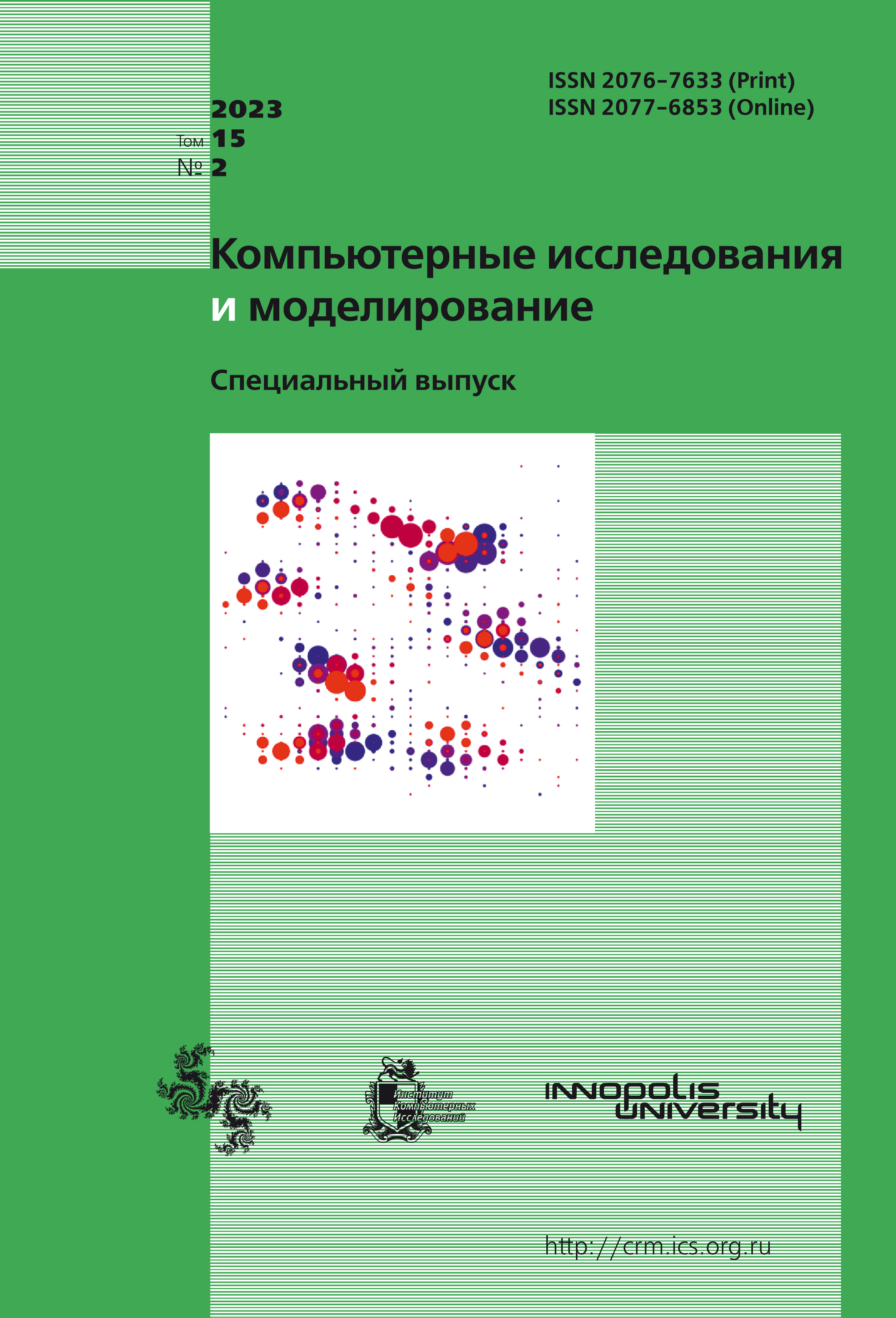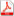Latest issue Issue 2, 2023 Vol. 15

# All issues

Comparsion of stochastic approximation and sample average approximation for saddle point problem with bilinear coupling termpdf (206K)

Stochastic optimization is a current area of research due to significant advances in machine learning and their applications to everyday problems. In this paper, we consider two fundamentally different methods for solving the problem of stochastic optimization — online and offline algorithms. The corresponding algorithms have their qualitative advantages over each other. So, for offline algorithms, it is required to solve an auxiliary problem with high accuracy. However, this can be done in a distributed manner, and this opens up fundamental possibilities such as, for example, the construction of a dual problem. Despite this, both online and offline algorithms pursue a common goal — solving the stochastic optimization problem with a given accuracy. This is reflected in the comparison of the computational complexity of the described algorithms, which is demonstrated in this paper.

The comparison of the described methods is carried out for two types of stochastic problems — convex optimization and saddles. For problems of stochastic convex optimization, the existing solutions make it possible to compare online and offline algorithms in some detail. In particular, for strongly convex problems, the computational complexity of the algorithms is the same, and the condition of strong convexity can be weakened to the condition of $\gamma$-growth of the objective function. From this point of view, saddle point problems are much less studied. Nevertheless, existing solutions allow us to outline the main directions of research. Thus, significant progress has been made for bilinear saddle point problems using online algorithms. Offline algorithms are represented by just one study. In this paper, this example demonstrates the similarity of both algorithms with convex optimization. The issue of the accuracy of solving the auxiliary problem for saddles was also worked out. On the other hand, the saddle point problem of stochastic optimization generalizes the convex one, that is, it is its logical continuation. This is manifested in the fact that existing results from convex optimization can be transferred to saddles. In this paper, such a transfer is carried out for the results of the online algorithm in the convex case, when the objective function satisfies the $\gamma$-growth condition.

Keywords: stochastic optimization, stochastic approximation, sample average approximation, decentralization
Citation in English: Skorik S.N., Pirau V.V., Sedov S.A., Dvinskikh D.M. Comparsion of stochastic approximation and sample average approximation for saddle point problem with bilinear coupling term // Computer Research and Modeling, 2023, vol. 15, no. 2, pp. 381-391
Citation in English: Skorik S.N., Pirau V.V., Sedov S.A., Dvinskikh D.M. Comparsion of stochastic approximation and sample average approximation for saddle point problem with bilinear coupling term // Computer Research and Modeling, 2023, vol. 15, no. 2, pp. 381-391
DOI: 10.20537/2076-7633-2023-15-2-381-391

Full-text version of the journal is also available on the web site of the scientific electronic library eLIBRARY.RU

The journal is included in the Russian Science Citation Index

The journal is included in the List of Russian peer-reviewed journals publishing the main research results of PhD and doctoral dissertations.

International Interdisciplinary Conference "Mathematics. Computing. Education"

The journal is included in the RSCI

Indexed in Scopus